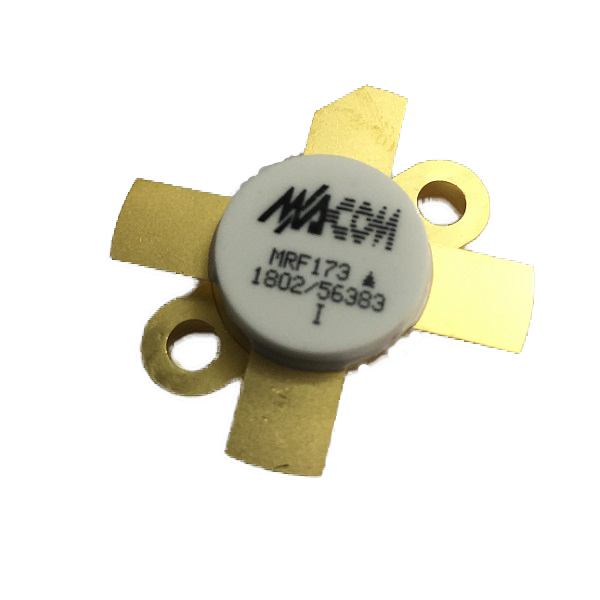# MRF173

•Liên hệ
Thương hiệu Khác
Mã sản phẩm

MACOM MRF173 MOSFET Transistor 80 W, 28 V, 175 MHz

FEATURES:

Designed for broadband commercial and military applications up to 200 MHz frequency range. The high-power, high-gain and broadband performance of  this device make possible solid state transmitters for FM broadcast or TV  channel frequency bands.

-          Guaranteed Performance at 150 MHz, 28 V

Output Power = 80 W

Gain = 11 dB (13 dB Typ)

Efficiency = 55% Min. (60% Typ)

-          Low Thermal Resistance

-          Ruggedness Tested at Rated Output Power

-          Nitride Passivated Die for Enhanced Reliability

-          Low Noise Figure — 1.5 dB Typ at 2.0 A, 150 MHz

-          Excellent Thermal Stability; Suited for Class A Operation

MAXIMUM RATINGS:

 Rating Symbol Value Unit Drain-Source Voltage VDSS 65 Vdc Drain-Gate Voltage VDGO 65 Vdc Gate-Source Voltage VGS ±40 Vdc Drain Current — Continuous ID 9.0 Adc Total Device Dissipation @ TC C°= 25 PD 220 Watts Derate above 25OC 1.26 W/oC Storage Temperature Range Tstg -65 to +150 oC Operating Temperature Range TJ 200 oC Thermal Resistance, Junction to Case RөJC 0.8 oC/W

ELECTRICAL CHARACTERISTICS   (TC = 25OC unless otherwise noted):

 Characteristic Symbol Min Typ Max Unit OFF CHARACTERISTICS: Drain-Source Breakdown Voltage (VDS = 0 V, VGS = 0 V)   ID = 50 mA V(BR)DSS 65 — — V Zero Gate Voltage Drain Current (VDS = 28 V, VGS = 0 V) IDSS — — 2.0 mA Gate-Source Leakage Current (VGS = 40 V, VDS = 0 V) IGSS — — 1.0 µA ON CHARACTERISTICS: Gate Threshold Voltage (VDS = 10 V, ID = 50 mA) VGS(th) 1.0 3.0 6.0 V Drain-Source On-Voltage (VDS(on), VGS = 10 V, ID = 3.0 A) VDS(on) — — 1.4 V Forward Transconductance (VDS = 10 V, ID = 2.0 A) gfs 1.8 2.2 — mhos DYNAMIC CHARACTERISTICS: Input Capacitance (VDS = 28 V, VGS = 0 V, f = 1.0 MHz) Ciss — 110 — pF Output Capacitance (VDS = 28 V, VGS = 0 V, f = 1.0 MHz) Coss — 105 — pF Reverse Transfer Capacitance (VDS = 28 V, VGS = 0 V, f = 1.0 MHz) Crss — 10 — pF FUNCTIONAL CHARACTERISTICS: Noise Figure (VDD = 28 V, f = 150 MHz, IDQ = 50 mA) NF — 1.5 — dB Common Source Power Gain(VDD = 28 V, Pout = 80 W, f = 150 MHz, IDQ = 50 mA) Gps 11 13 — dB Drain Efficiency (VDD = 28 V, Pout = 80 W, f = 150 MHz, IDQ = 50 mA) η 55 60 — % Electrical RuggednessVDD = 28 V, Pout = 80 W, f = 150 MHz, IDQ = 50 mA) Load VSWR 30:1 at all phase angles Ψ No Degradation in Output Power Series Equivalent Input Impedance(VDD = 28 V, Pout = 80 W, f = 150 MHz, IDQ = 50 mA) Zin - 2.99–j4.5 - Ω Series Equivalent Output Impedance(VDD = 28 V, Pout = 80 W, f = 150 MHz, IDQ = 50 mA) Zout - 2.68–j1.3 - Ω